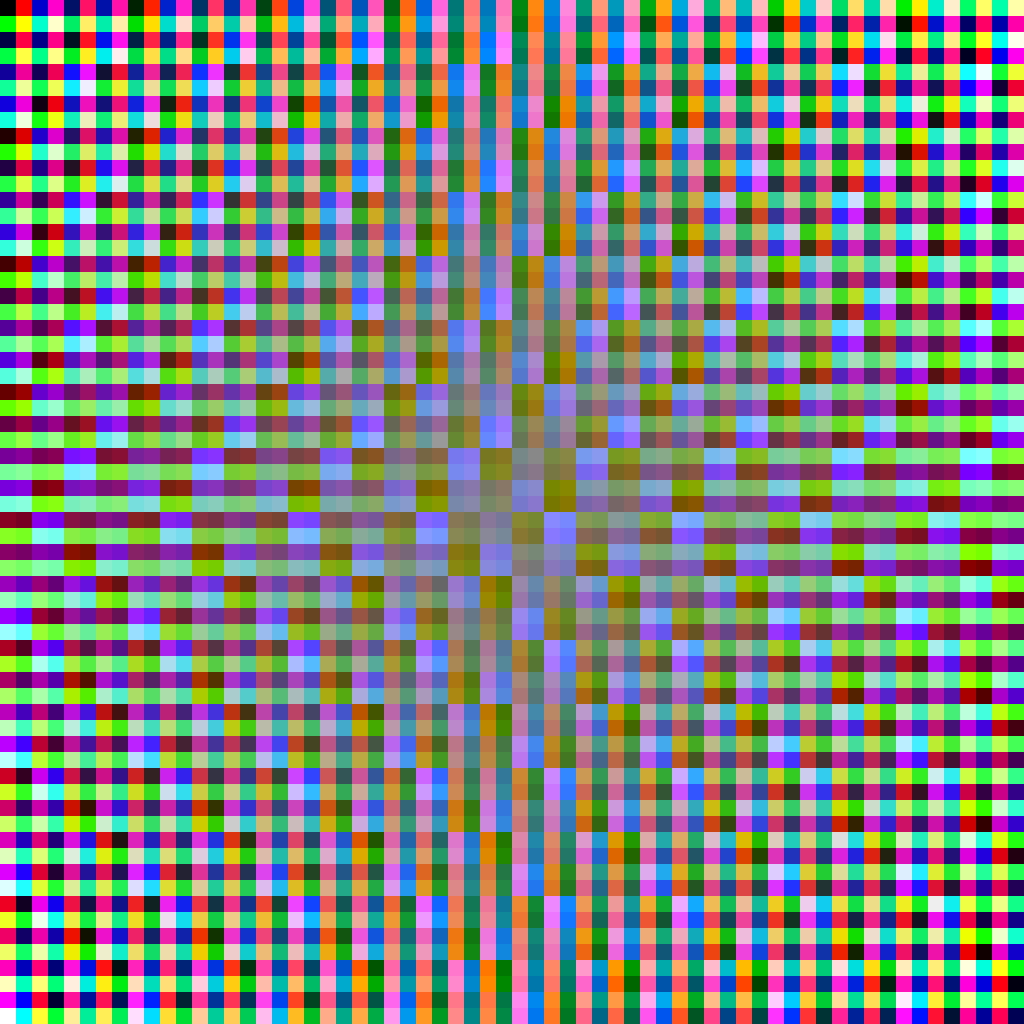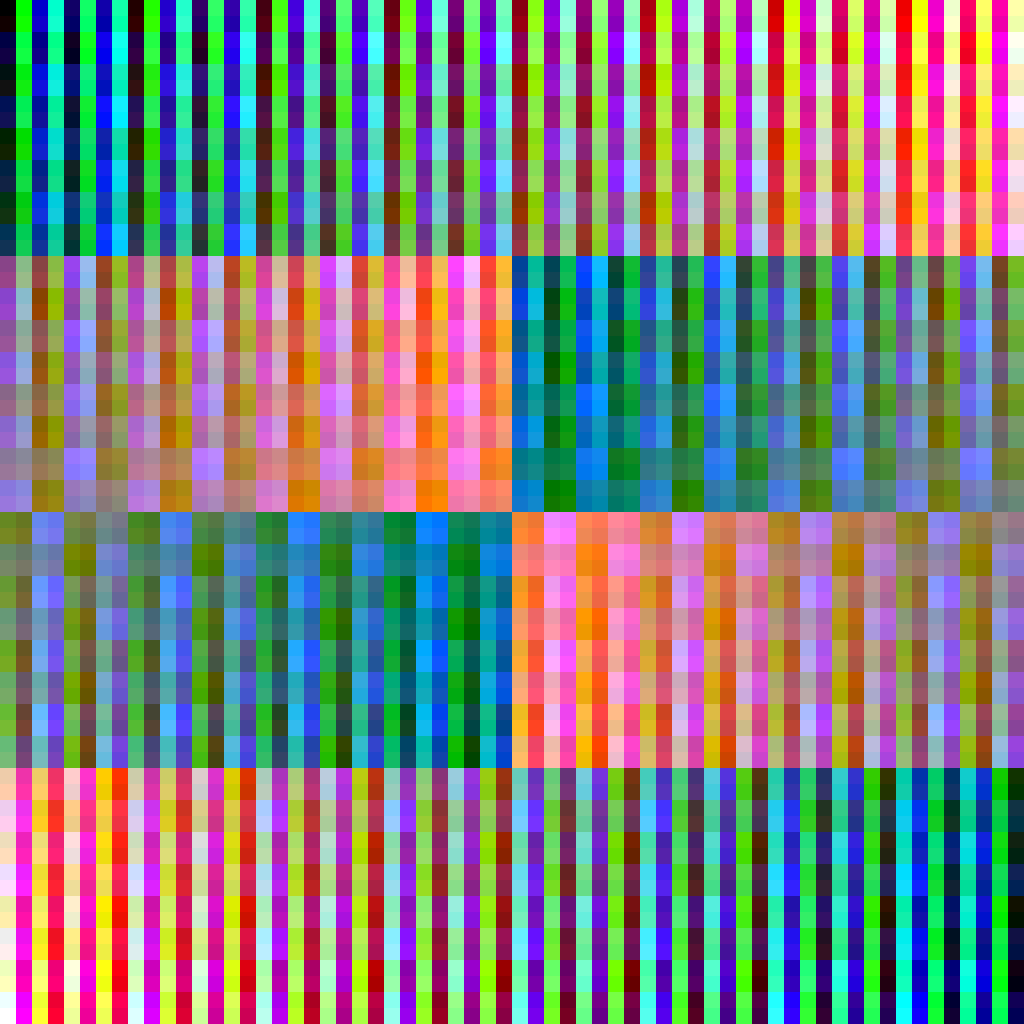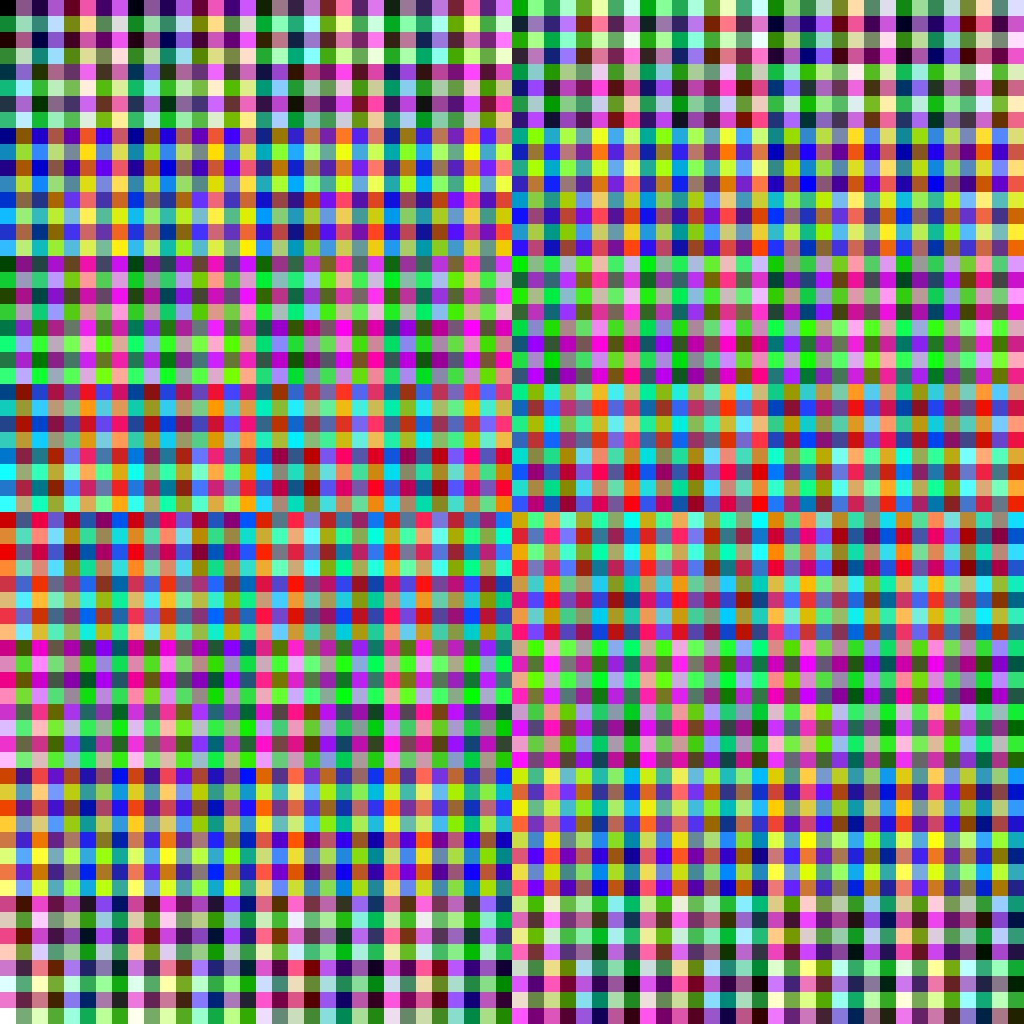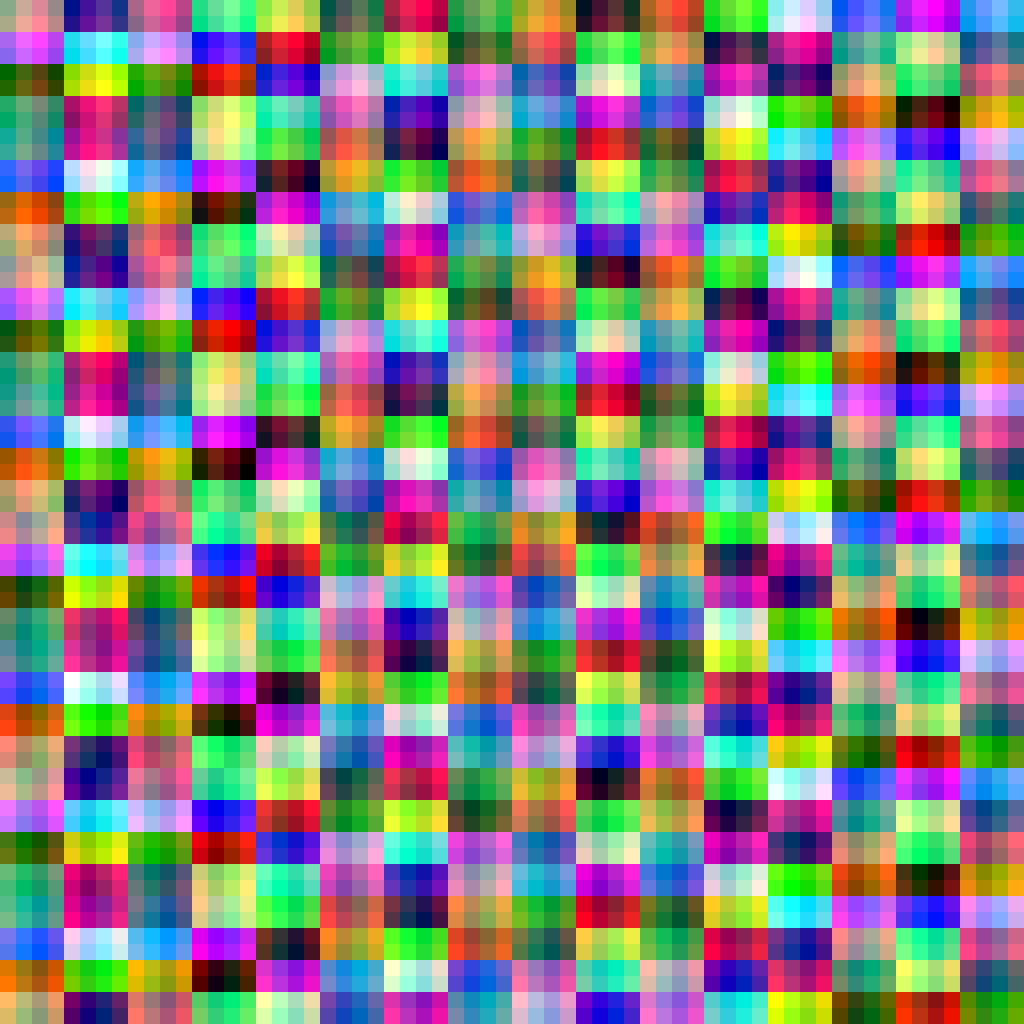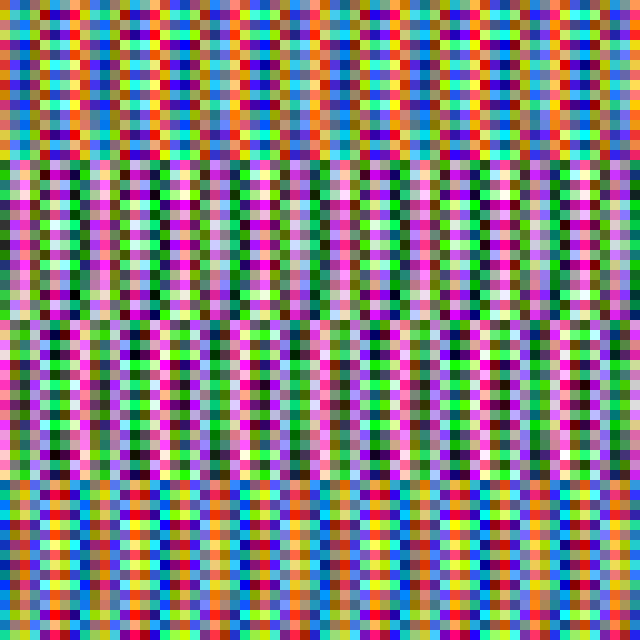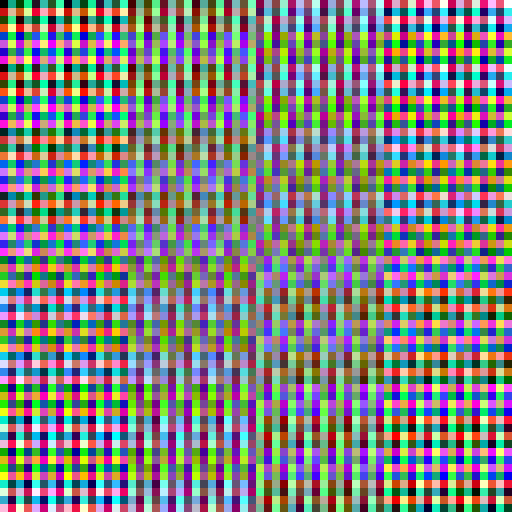# Colored Magic Squares

2014

A magic square is a grid filled with ascending numbers such that the sum of each row, column and diagonal is equal. Below you see an example square with order 4:I found a way of constructing perfect magic squares from binary numbers. I'm not sure if somebody else invented that before, but I couldn't find something similar. If you do, please let me know. With that method it's quite easy to create magic squares with orders that are powers of 2: 4, 8, 16, 32, and so on. Let's make an example with a 4 x 4 square.

First we need to have a set of binary layers. Each of these layers is a grid of 4 x 4 where each cell is either zero or one (in most of the images here I just use white and black to symbolize 0 and 1). These zeroes and ones must be perfectly distributed: each row, column and diagonal must contain the same amount of zeroes and ones.

I found the following type of layers quite useful. I call them long-checkered because its like a checkerboard pattern but stretched in one direction.For squares with order 4 there are only eight different long-checkered layers possible. Above you see four of them. The other four are just inverted versions of these.A square of order 4 has 16 cells. Therefore we need 16 different numbers. 16 is 24. So, why not combining four layers and read the cells at the same position as a binary number. With the four layers shown above, the result would look like that.Of course, the numbers here are from 0 to 15 instead from 1 to 16 what would be considered the usual range for a magic square. But that's not really a difference.

There is a bigger problem. Not every combination of 4 layers will produce all 16 numbers. With this square of order 4 it might not be to difficult to find a rule for which layers could be combined. It's quite obvious for example, that you can't combine a a layer with its inverted version. For the bigger squares to come, I couldn't find a simple rule. I had to use trial and error at that point. If you have suggestions, you're welcome!

The great thing is, that the resulting magic square is really good, because it does not only fit the minimum conditions for a magic square: same sums for each row, column and diagonal. It is also a pandiagonal magic square and a most-perfect magic square. This is because each offset diagonal, each 2×2 sub-square and the corners of each 3×3 sub-square sum up to the same number.## Extending and coloring

My initial goal was to create a colored version of a magic square. There are many ways of translating a number into a color. In most color models a color is defined by three values. For color on screen the three values are the red, green and blue component of the color.

So, I needed to split up one number into three. One of the easiest ways (and as it turned out, a very good way for my purpose) is to take that number in binary representation and split it up in three groups. A four digit binary number isn't a good start for doing such a split. What about six digits? 26 is 64, which is good because 64 is a square number. This will lead to a 8 x 8 square.

#### Translation to a color

An example:

• Dezimal number → 40
• Binary representation → 101100
• Splitting up → 10 11 00
• Dezimal again → 2 3 0
• Multiplied by 85 → 170 255 0
• Color → red = 170, green = 255, blue = 0

And that's the result when doing this with all the numbers in the square.

Click on the square below to produce new random versions.

## 4096 colors

The 8 x 8 square is cool, but does not reveal much of the numerical structure it is build of. In most cases it looks much like a square of random colors.

The next square order to try is 64. Why this? The amount of digits in my binary numbers (which is the same as the number of layers) should be a multiple of 3. Otherwise red, green and blue will not be balanced out. We already use 6 layers in the example above.

• 6 layers → 26 = 64 cells → 8 x 8 square
• 9 layers → 29 = 512 cells → 512 is not a square number
• 12 layers → 212 = 4069 cells → 64 x 64 square

Nine layers will not work, that's why I chose the 64 x 64 square. There are 30 possibilities of long-checkered binary layers when ignoring all possible offsets. Below I show 15, all of those could be rotated by 90 degrees: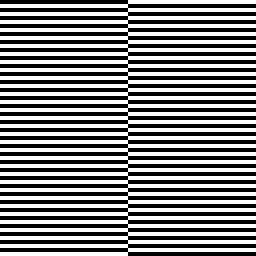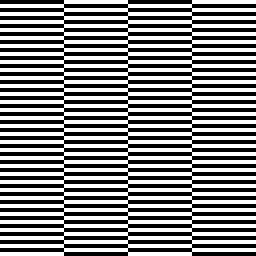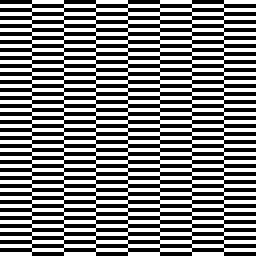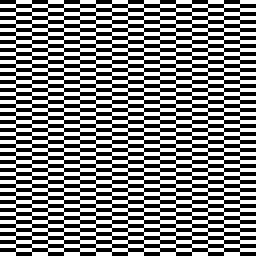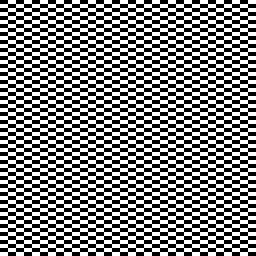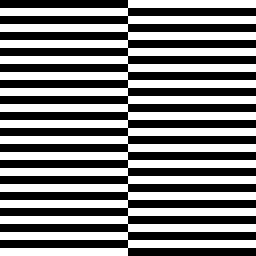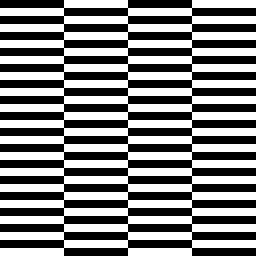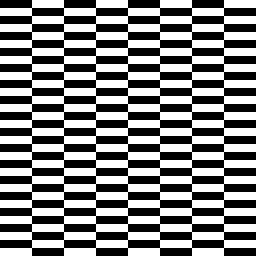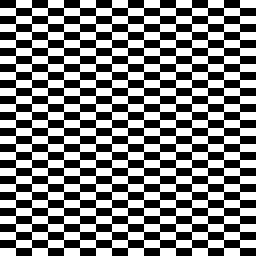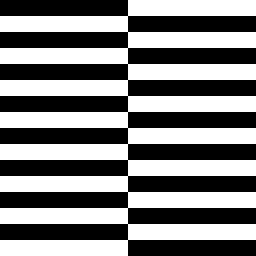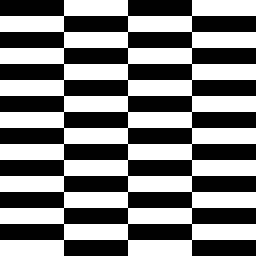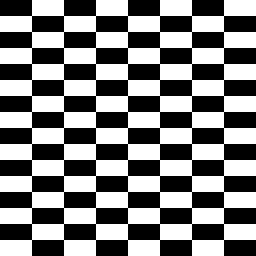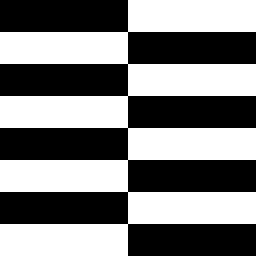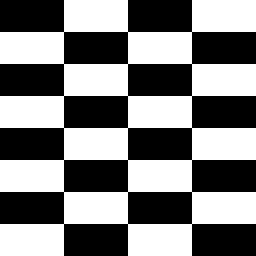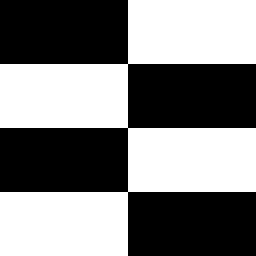I used only the first 12 and its rotated versions, because they produce most-perfect magic squares where every little 8 x 8 square sum up to the same number.

And that's what we get here. Click on the square below to produce new random versions

## Play around

I've also prepared a version of this programm where you can edit the layer parameters in detail. Just follow the link here:

## Some examples